# Raspberry Pi_Eng_23.5.3 Python Syntax

#### Published Book on Amazon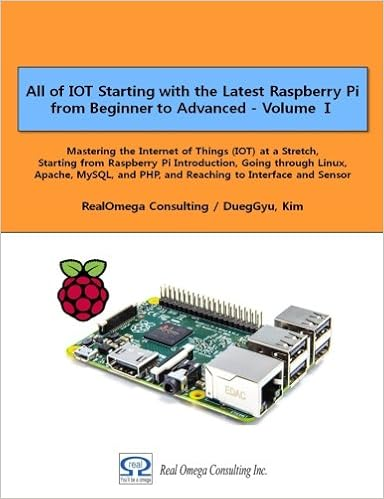All of IOT Starting with the Latest Raspberry Pi from Beginner to Advanced – Volume 1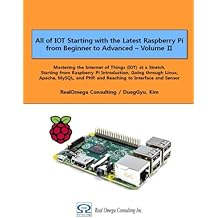All of IOT Starting with the Latest Raspberry Pi from Beginner to Advanced – Volume 2

#### 출판된 한글판 도서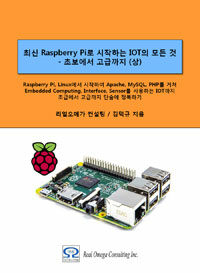최신 라즈베리파이(Raspberry Pi)로 시작하는 사물인터넷(IOT)의 모든 것 – 초보에서 고급까지 (상)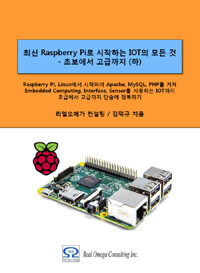최신 라즈베리파이(Raspberry Pi)로 시작하는 사물인터넷(IOT)의 모든 것 – 초보에서 고급까지 (하)

### 23.5.3Python Syntax

#### 23.5.3.1Starting Statement of Python

When writing a Python program, it is a good idea to start with "#!" (shebang). This line tells the operating system where to look for Python files. This line is not needed when executing a program within IDLE or calling "python' command separately on Terminal, and it is necessary if the program file name is directly called and executed. It is used to allow programs to be executed regardless of where executable files of program written in Python are installed.

The first line of a Python program usually begins with the following sentence. This means that it refers to environment variable "\$ PATH" with command "/usr/bin/env" to find the location of Python program and execute it

 `#!/usr/bin/env  python`

This line tells Raspberry Pi system to refer to "\$PATH" environment variable so that the operating system can work without problems when locating Python, no matter what Linux distribution is used. "\$PATH" variable contains a list of directory in which executable files are stored, and is used to locate the program when users hit program names to run on the Console or Terminal screen.

Some Python program begins with the following sentence, which means to use the program in "/usr/bin/python" directly without looking for the location of program using environment variable.

 `#!/usr/bin/python`

#### 23.5.3.2Simple Printing

To print "Hello world" in Python, use command as follows. It is very simple.

 `print("Hello world")`

#### 23.5.3.3Indentation of Program

In development languages like C, the several rows that are contained together inside is grouped together into one bundle by using braces { }, and the indentation for each row that is used to visually represent internal inclusions is freely arranged by the developer. However, Python does not use braces { } to represent internal inclusions, but instead strongly requires indentation. Indenting not only aligns the visual appearance, but also affects the execution of the program.

Let's look at the differences between C program and Python program below. First, in C language, by using indentation, program code can be arranged to be read well, or it can be compressed easily, so it makes it possible to arrange various types of sentences.

 `// C program indented well``int factorial(int x)``{``    if(x == 0) {``        return 1;``    } else {``        return x * factorial(x - 1);``    }``}`` ``//  C program written hard to read``int factorial(int x) { `` if(x == 0) {return 1;} else`` {return x * factorial(x - 1); } }`

Here is a Python program with the same contents as the previous C program. In Python, it is not allowed to arrange it in various ways, as in C, and it is required to do a certain pre-defined type of indentation.

 `def factorial(x):``    if x == 0:``        return 1``    else:``        return x * factorial(x - 1)`

The following is an example of using loop processing for iteration in Python.

 `for i in range(10):``    print("Hello")`

In the above, indentation is essential. The second line indented is part of loop processing statement. If the second line is not indented, it means that it is outside the loop processing. Consider the following example. When you run this code, both "print" statements are treated as if they are inside the loop, resulting in the following results.

 `for i in range(2):``    print("A")``    print("B")`

 `A``B``A``B`

Now let's leave the content as it is, just adjust the indentation only, and try to run again.

This time "print ("A")" statement is inside the loop, and "print ("B")" is treated as outside the loop, resulting in another result.

 `for i in range(2):``    print("A")``print("B")`

 `A``A``B`

The following example is for indentation in "if" statement. You can see that statements to be processed by unit of block are arranged in indentation so that two "print" statements are executed when "True", and another two "print" statements are executed when "False".

 `if True:``    print("Answer")``    print("True")``else:``    print("Answer")``    print("False")   `

#### 23.5.3.4Using Variable

You can use variable when you want to temporarily store a certain value in a program. To store a value to a variable, use it as follows.

 `name = "Bob"``age = 15`

In the previous, data type is not specified in advance for these variables. In Python, the data type is self-determined by guessing, and it can be changed dynamically.

 `age = 15``age += 1  # increment age by 1``print(age)`

Comments are ignored when program is run. Comments are used to keep a record of program writer or a lot of information that other person can refer to later. You can use "#" (hash) symbol to indicate comments. It can start at the beginning of sentence, or at the end of sentence. If you want to indicate multiple lines of comments, you can use """  """ (triple quote). The following code is an example of using comments

 `# this test program``age = 15``print(age)     # age is printed``"""``This is a very simple Python program that prints "Hello".``That's all it does.``"""``print("Hello")`

#### 23.5.3.6List

In Python, "list" is used to represent data collection of all data types. It is called "array" in other language. Use "[  ]" (square bracket) to represent "list", and separate items inside with "," (comma).

 `numbers = [1, 2, 3]`

#### 23.5.3.7Iteration Processing

Data of a certain data type can be iterable. This means that loop processing is possible over the datas contained within. Here is an example of a list. This prints out the value for each item contained in the list "numbers".

 `numbers = [1, 2, 3]`` ``for number in numbers:``    print(number)`

 `1``2``3`

Notice that "number" is used to represent each item in the list "numbers". In Python, the use of words that describe the nature of a variable is recommended. It is recommended to use plural word for list and singular word for item. This makes it easy to understand when you read it later.

There are other data types that can be iterated. For example, "string" data. The following code prints iteratively for each character of a word stored in "dog_name".

 `dog_name = "BINGO"`` ``for char in dog_name:``    print(char)`

 `B``I``N``G``O`

#### 23.5.3.8"range" Statement

Integer data type can not be iterated. If you try to iterate for such data, an error occurs. For example, let's run the following code: Then the following error will occur.

 `for i in 3:``    print(i)`

 `TypeError: 'int' object is not iterable`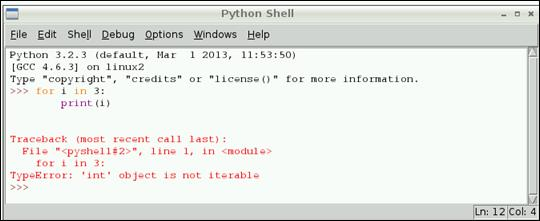However, by using "range" function, you can create an object that can be iterated. Consider the following example. Here, "range(3)" means three numbers from 0 to 2. "range(5)" means the number 0, 1, 2, 3, 4 (all 5 numbers). If you need numbers 1 through 5, use "range (1, 5)".

 `for i in range(3):``    print(i)`

#### 23.5.3.9"len" Statement

If you want to know the size of "string" or "list", you can use "len" function.

 `name = "Jamie"``print(len(name))  # print 5`` ``names = ["Bob", "Jane", "James", "Alice"]``print(len(names))  # print 4`

#### 23.5.3.10"if" Statement

If you want to control the  processing flow of program, use "if" statement.

 `name = "Joe"`` ``if len(name) > 3:``    print("Nice name,")``    print(name)``else:``    print("That's a short name,")``    print(name)`

#### 23.5.3.11Loop processing

If you want to execute a specific task repeatedly, you can use loop processing.

"while" statement

"while" statement performs specified operations repeatedly until a certain condition is satisfied.

In the following example, if user enters "hello", it stops processing.

 ` n = raw_input("Please enter 'hello':")``while n.strip() != 'hello':``    n = raw_input("Please enter 'hello':")`

"for" statement

"for" statement performs a specified operations for a specified range.

The example below prints each word and its length stored in the "words" variable.

 `# Measure some strings:``words = ['cat', 'window', 'defenestrate']``for w in words:``    print(w, len(w))`